3 篇文章 11 订阅

十大经典排序算法-堆排序,计数排序,桶排序,基数排序

前言1-堆排序

• 二叉树• 完全二叉树• 大根堆• 小根堆

• 1.将数组重构成大根堆

• 2.将数组的队头元素与队尾元素交换位置

• 3.对去除了队尾元素的数组进行重构,再次重构成大根堆)))

• 堆排序是不稳定的,这个其实还是比较好理解的.因为我们在进行小分支的调整时时有序的,但是之后可能会出现小分支扩大到大分支进行重新的调整,那么这时候就有可能会出现元素的相对位置混乱的情况.

这个混乱的过程其实有点像我们之前所说的希尔排序,希尔排序也是小区间内的插入排序是有序的,但是一旦扩散到更大的区间进行二次的插入排序时就有可能造成相对位置混乱的情况.

说的差不多了,那么我们接下来还是举一个例子来帮助大家更好的理解:通过上面这张图,相信大家就能更好的理解堆排序为啥是不稳定的了.

• 堆排序每轮排序都可以确定一个元素的最终位置,这个大家看我上面的演示图也能看出来.//交换数组中的元素
public static void swap(int[]num ,int i,int j) {
int temp=num[i];
num[i]=num[j];
num[j]=temp;
}
//将待排序的数组构建成大根堆
public static void buildbigheap(int []num,int end) {
//从最后一个非叶子节点开始构建,依照从下往上,从右往左的顺序
for(int i=end/2;i>=0;i--) {
int left=2*i+1;
int right=2*i+2;
int big=i;
//判断小分支那个是大元素
if(left<end&&num[i]<num[left])
//				swap(num, i, left);
i=left;
if(right<end&&num[i]<num[right])
//				swap(num, i, right);
i=right;
swap(num, i, big);
}
}
public static void main(String[] args) {
int []num ={7,4,9,3,2,1,8,6,5,10};
long startTime=System.currentTimeMillis();
//第一次构建大根堆
buildbigheap(num, num.length);
for(int j=num.length-1;j>0;j--) {
//交换队头已经排序得到的最大元素与队尾元素
swap(num, 0, j);
System.out.print("第"+(num.length-j)+"次排序:  ");
for(int k=0;k<num.length;k++) {
System.out.print(num[k]+" ");
}
System.out.println();
//交换结束之后,大根堆已经内破坏,需要开始重新构建大根堆
buildbigheap(num,j);
}
long endTime=System.currentTimeMillis();
System.out.println("程序运行时间： "+(endTime-startTime)+"ms");
}//交换数组中的元素
public static void swap(int[]num ,int i,int j) {
int temp=num[i];
num[i]=num[j];
num[j]=temp;
}
//将待排序的数组构建成大根堆
public static void buildbigheap(int []num,int end) {
//从最后一个非叶子节点开始构建,依照从下往上,从右往左的顺序
for(int i=end/2;i>=0;i--) {
}
}
//调整该节点及其以下的所有节点
public static void  adjustnode(int i,int end,int []num) {
int left=2*i+1;
int right=2*i+2;
int big=i;
//判断小分支那个是大元素
if(left<end&&num[i]<num[left])
i=left;
if(right<end&&num[i]<num[right])
i=right;
if(i!=big) {
//交换顺序之后需要继续校验
swap(num, i, big);
//重新校验,防止出现交换之后根节点小于孩子节点的情况
}
}
public static void main(String[] args) {
int []num ={5,3,7,1,4,6,2};
long startTime=System.currentTimeMillis();
//第一次构建大根堆
buildbigheap(num, num.length);
for(int j=num.length-1;j>0;j--) {
System.out.print("第"+(num.length-j)+"次排序前:  ");
for(int k=0;k<num.length;k++) {
System.out.print(num[k]+" ");
}
//交换队头已经排序得到的最大元素与队尾元素
swap(num, 0, j);
System.out.print("第"+(num.length-j)+"次排序后:  ");
for(int k=0;k<num.length;k++) {
System.out.print(num[k]+" ");
}
System.out.println();
//交换结束之后,大根堆已经被破坏,需要开始重新构建大根堆
buildbigheap(num,j);
}
long endTime=System.currentTimeMillis();
System.out.println("程序运行时间： "+(endTime-startTime)+"ms");
}• 时间复杂度

堆排序的本质思想也是利用了二叉树的特性,所以根据他的遍历次数以及二叉树的层数可以得到堆排序的时间复杂度为O(N*logn),不仅仅是平情况是这样最好与最坏的情况都是如此.

• 空间复杂度

这个我们可以看到我们整个排序的过程中只增加一个存储交换元素的temp,所以堆排序的空间复杂是常量级别的仅为O(1).

2-计数排序

• 1.第一次遍历序列,找出序列中的最大值以及最小值,然后根据最大值MAX最小值MIN创建一个MAX-MIN+1长度的数组.为什么创建这样长度的数组呢,因为只有创建了这样长度的数组,MIN-MAX区间内的每个元素才有对应的位置进行存放,如下图所示: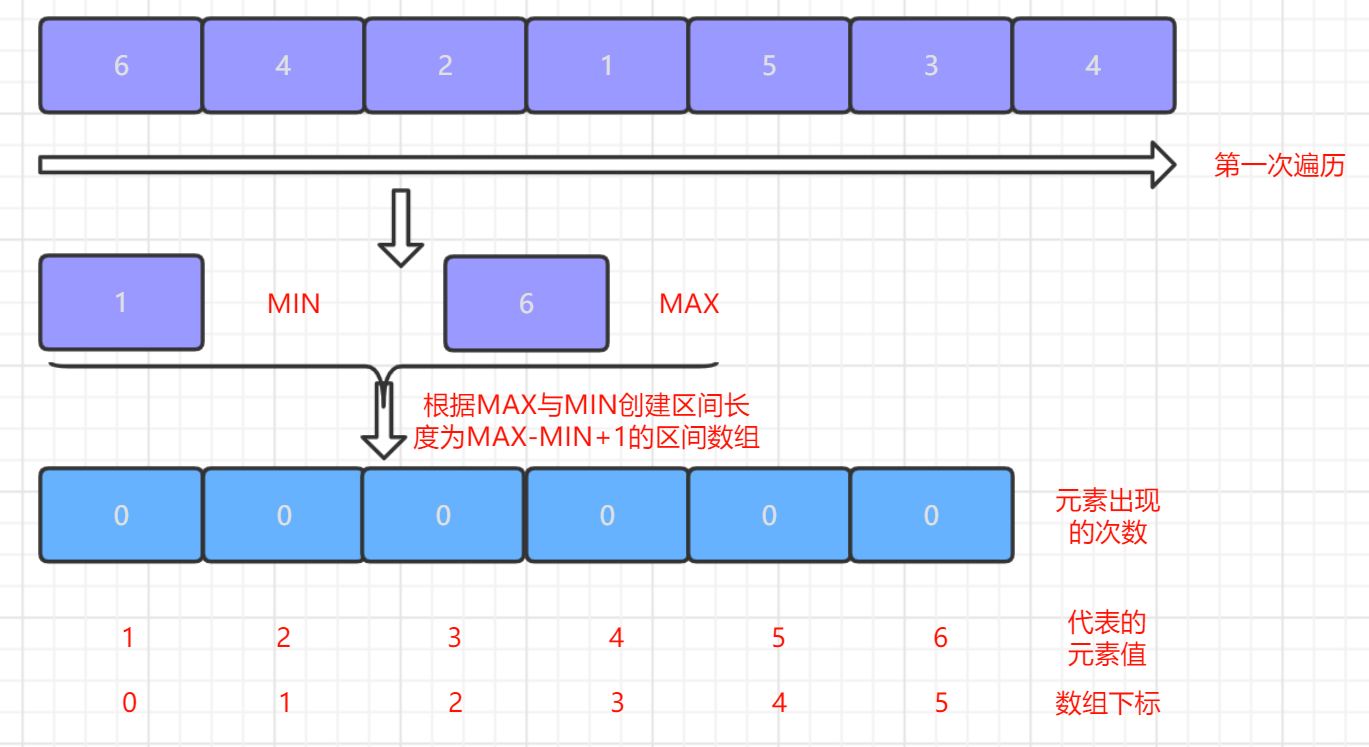• 2.第二次遍历序列,我们每次遍历一个元素都将该元素所对应的区间数组对应的位置进行+1操作,这个步骤其实就是我们计数排序的核心----计数了.遍历结束之后,区间数组中的元素值就代表相应元素出现的次数,如下图所示: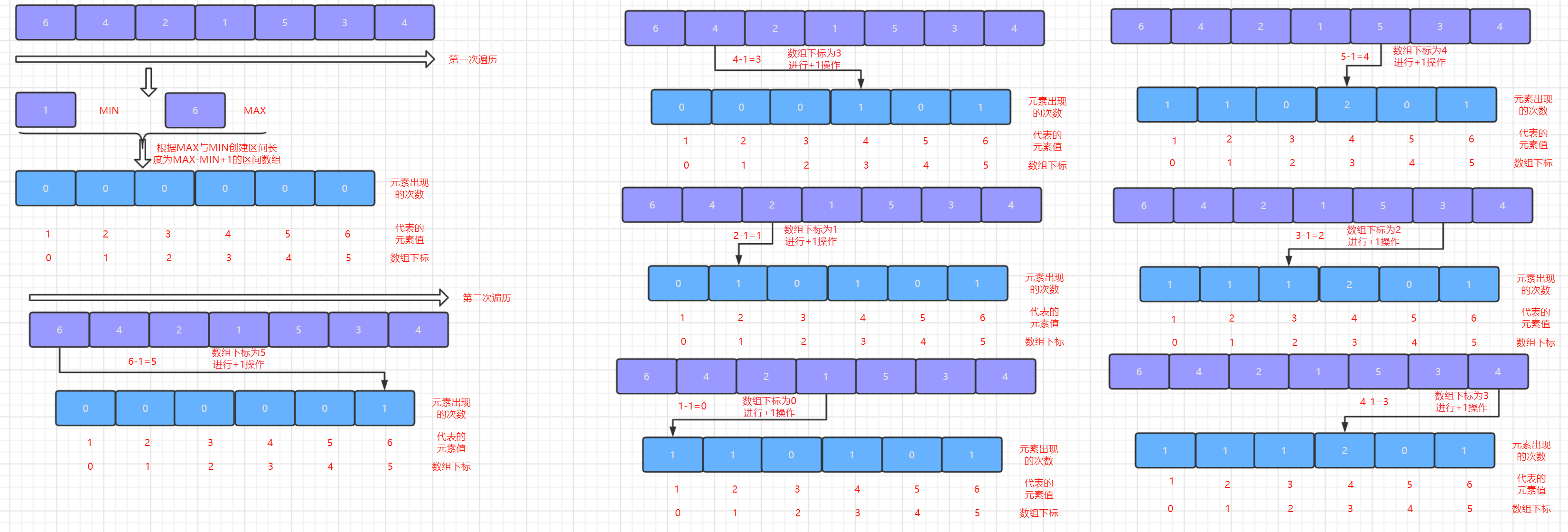• 3.最后一步就只需要按照区间数组中的次数一次将该元素打印出来即可.如下图所示: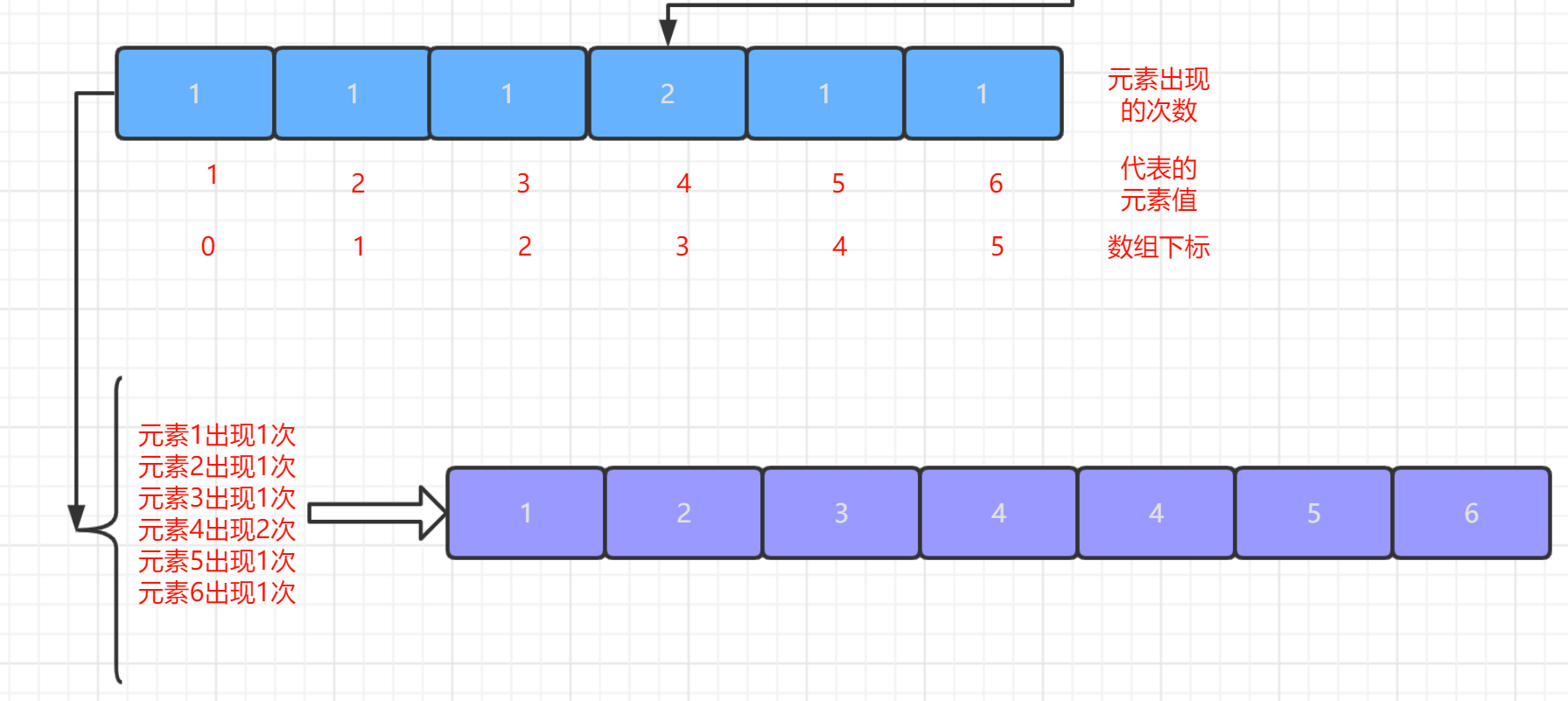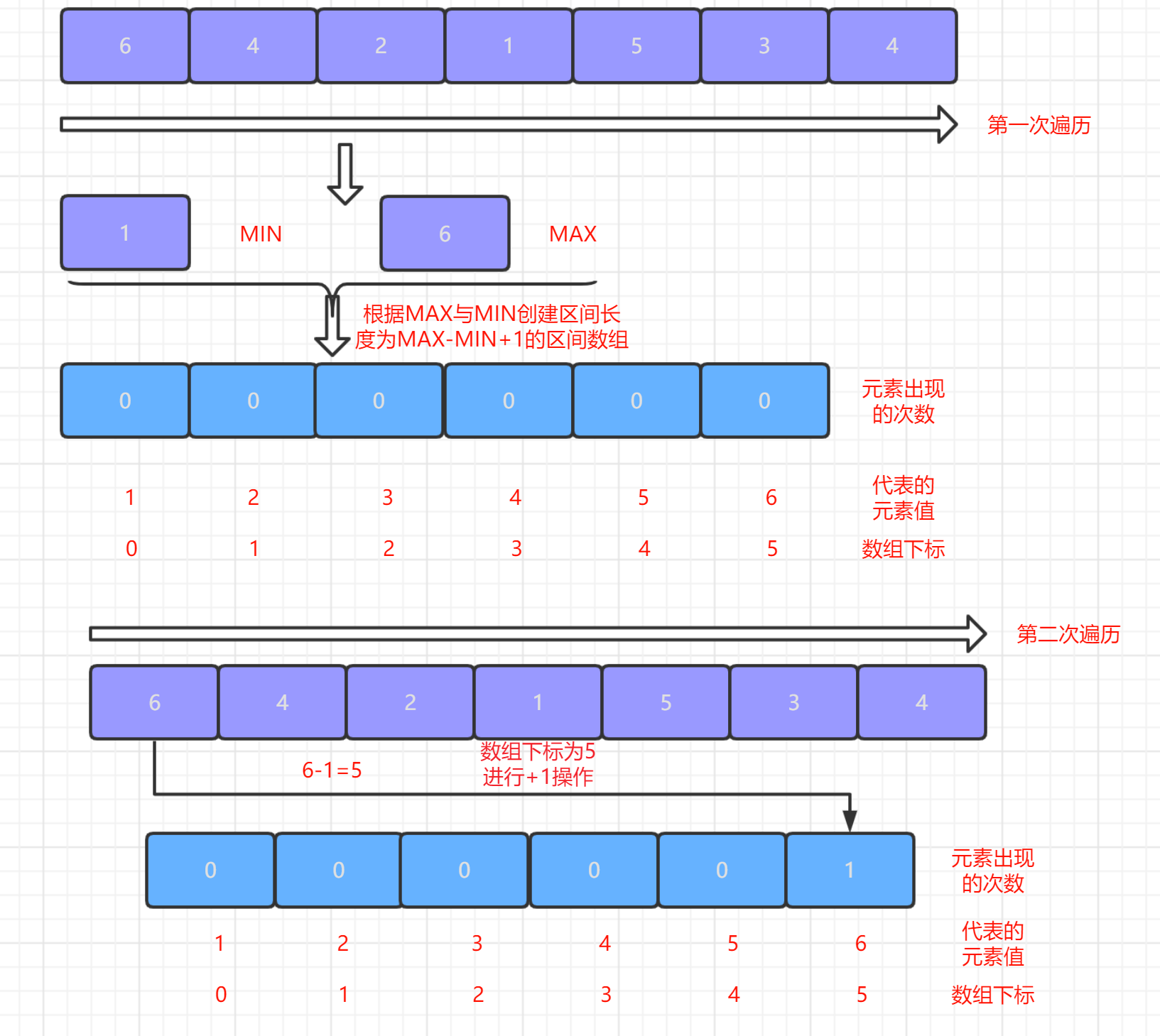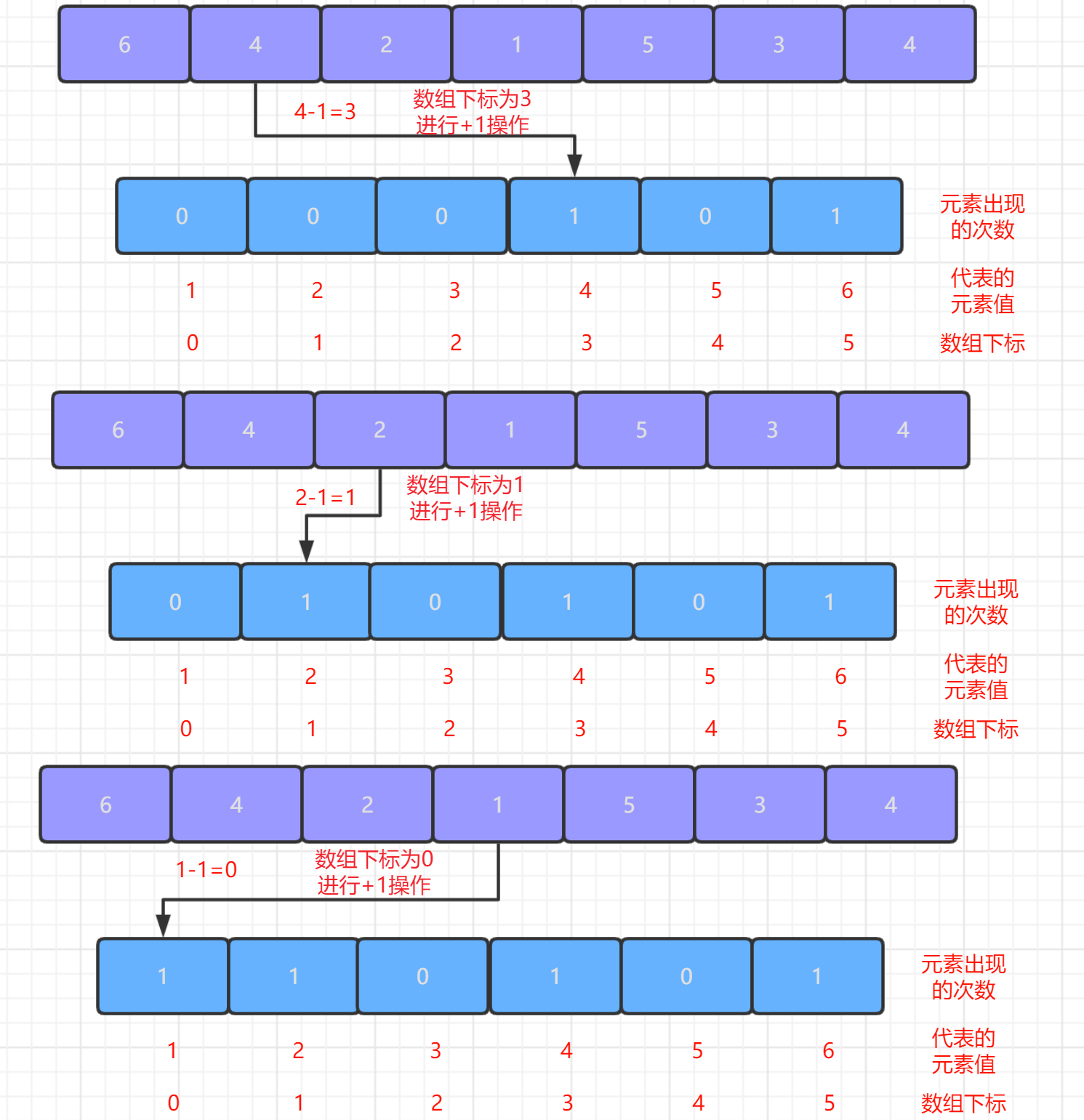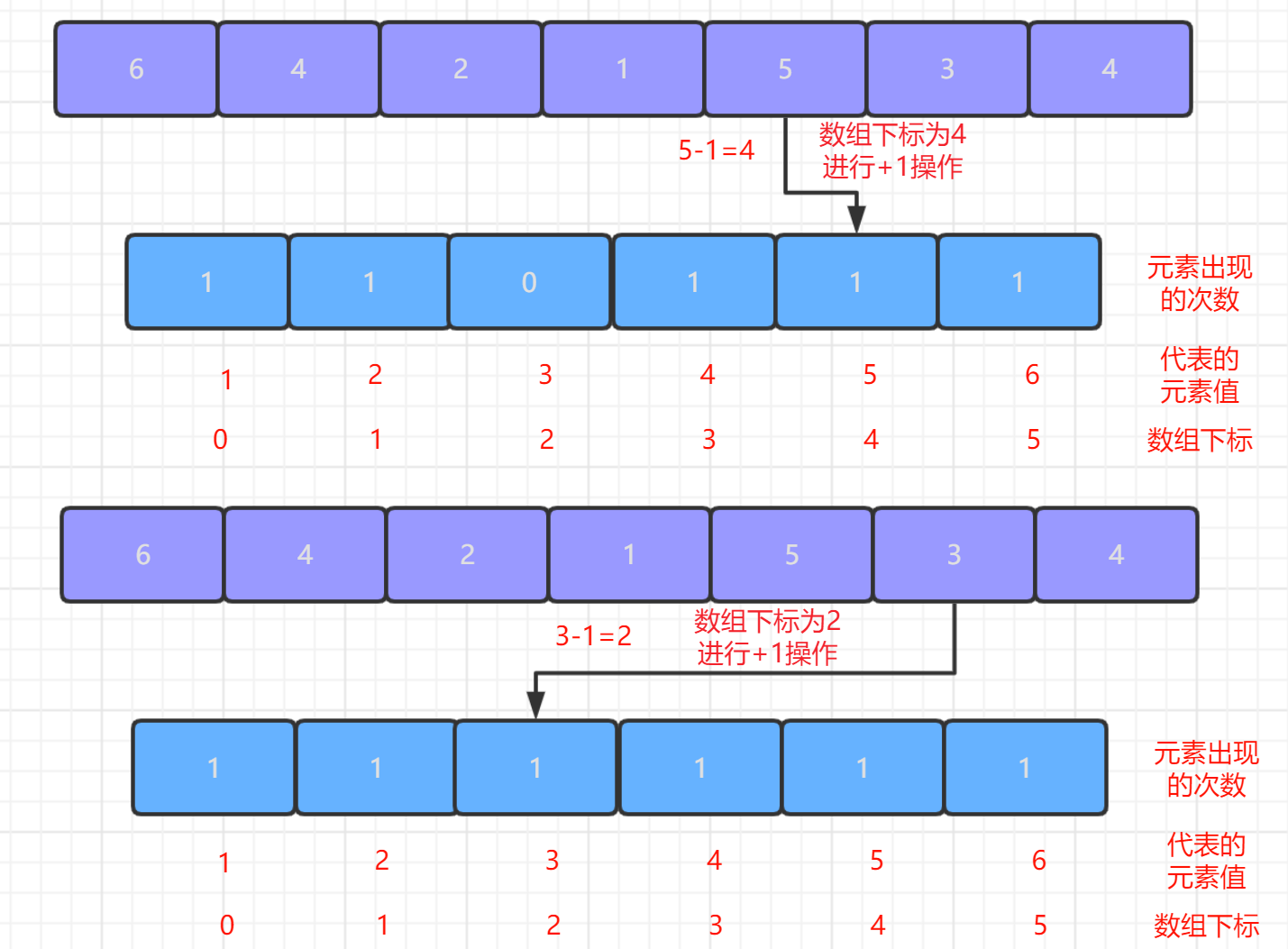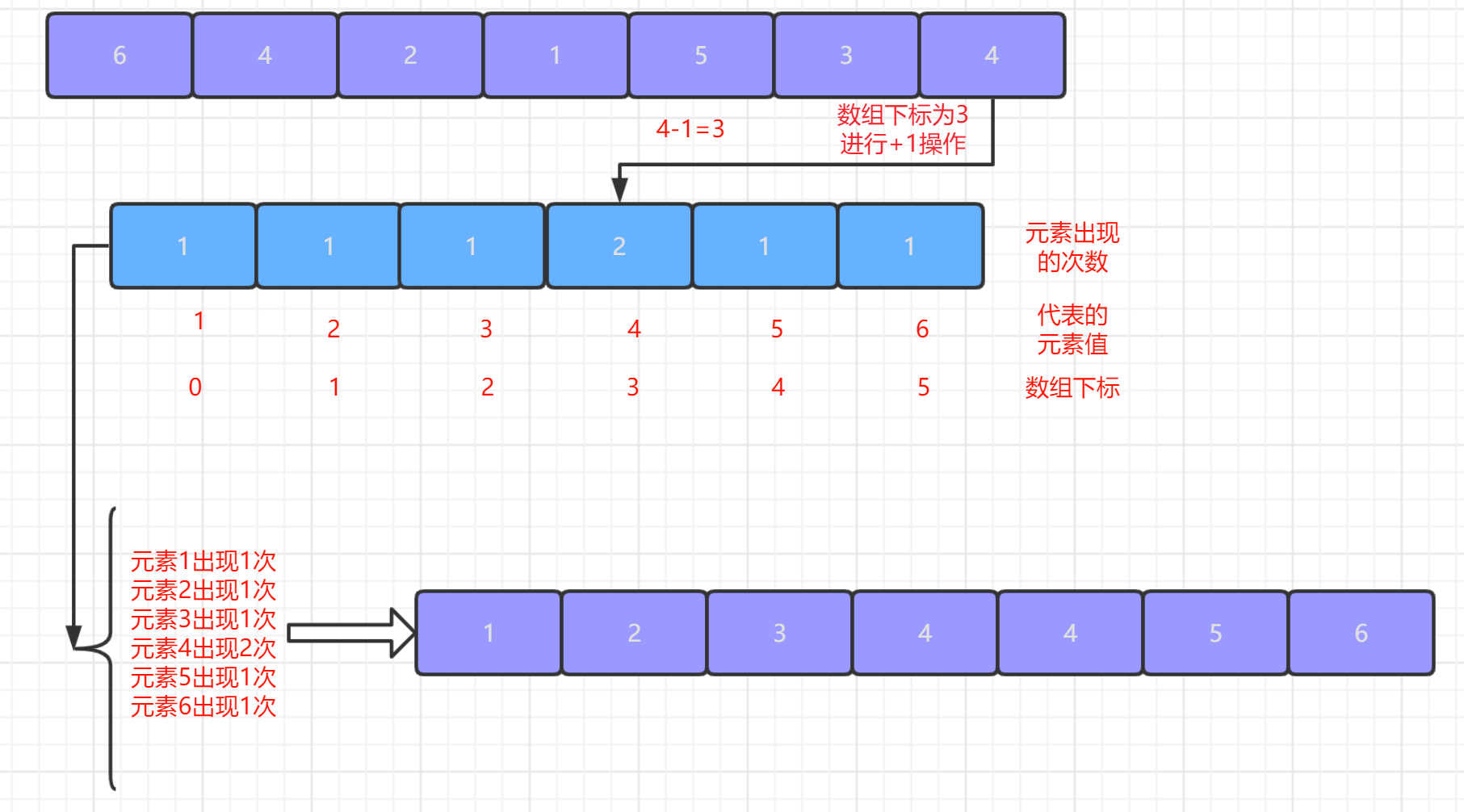-计数排序是稳定的 ,这个大家应该能很明显的看出来,因为计数排序本身并不是基于比较的算法.

-计数排序需要的额外空间比较大,这个大家很明显的看出来,并且空间浪费的情况也会比较严重,因为一旦序列中MAX与MIN的差距过大,那么需要的内存空间就会非常大.并且假如序列中的元素都是散布在一个特定的区间内,那么内存空间浪费的情况也会非常明显.public static void main(String[] args) {
int []num ={7,4,9,3,2,1,8,6,5,10};
long startTime=System.currentTimeMillis();
int min=Integer.MAX_VALUE;
int max=Integer.MIN_VALUE;
//先找出数组中的最大值与最小值
for(int i=0;i<num.length;i++) {
if(num[i]<min)
min=num[i];
if(num[i]>max)
max=num[i];
}
//创建一个长度为max-min+1长度的数组来进行计数
int []figure=new int [max-min+1];
for(int i=0;i<num.length;i++) {
//计算每个数据出现的次数
figure[num[i]-min]++;
}
int begin=0;
//创建一个新的数组来存储已经排序完成的结果
int []num1=new int [num.length];
for(int i=0;i<figure.length;i++) {
//循环将数据pop出来
if(figure[i]!=0) {
for(int j=0;j<figure[i];j++) {
num1[begin++]=min+i;
}
}
}
System.out.println("数据范围:"+min+"~"+max);
System.out.println("计数结果:  ");
for(int i=0;i<num.length;i++)
System.out.println("         "+num[i]+"出现"+figure[num[i]-min]+"次");
System.out.print("排序结果:  ");
for(int i=0;i<num1.length;i++)
System.out.print(num1[i]+"   ");
System.out.println();
long endTime=System.currentTimeMillis();
System.out.println("程序运行时间： "+(endTime-startTime)+"ms");
}• 时间复杂度

计数排序很明显是一种通过空间来换时间的算法,因为我们可以很明显的看到计数排序需要三次遍历,两次遍历我们的原序列,第三次是遍历我们的区间数组.那么很明显时间复杂度一定是线性级别的但是因为第三次遍历的并不是我们的原序列,而是我们的区间数组,所以时间复杂度并不是我们的平常的O(n),而是应该加上我们遍历区间数组的时间,假设我们的区间数组长度为k的话,那么我们的时间复杂度就是O(n+k)

• 空间复杂度

上面我们已经说过了,计数排序本身就是一个通过空间来换取时间的算法,所以很明显他的空间复杂度就会很高.并且这个空间复杂度主要就取决于我们区间数组的长度,所以假设我们的区间数组长度为k的话,那么我们的空间复杂度就为O(k)

3-桶排序• 1.我们首先需要第一次遍历我们的序列,得到我们序列中的最大值MAX以及序列中的最小值MIN,找到我们序列中的最大值与最小值之后,那么我们就可以确定序列中的所有都是在MIN~MAX这个数据范围区间之中.

• 2.第二步我们就是需要根据序列的数据范围来确定我们到底需要几个桶来存放我们的元素,这一步其实是比较关键的,因为桶的数量太多或者太少都会降低桶排序的效率.

我们举两个例子:

假设我们桶的数量太少,就比如说只有一个桶:那么很显然我们的桶排序就又重新退化成我们前两篇内容里介绍的比较算法了.

又假设我们桶的数量太多,就比如说有MAX-MIN+1个桶:那么很显然这时候的桶排序又重新退化成了我们上面刚刚讲解过的计数排序了.

所以说我们需要确定好一个适中的桶的数量,不然回就会出现我们上面所说到的几种情况.但是有没有一个特定的公式来确定桶的数量.所以我们还是只能自己确定桶的数量.但是有一个规则我们还是可以考虑进去的,那就是最好让元素平均的分散到每一个桶里.

• 3.确定完桶的数量之后,我们就可以给每个桶来划分数据范围了.一般是这样划分的,(MAX-MIN+1)/桶的数量,得到的结果就是桶长.之后每个桶的数据范围就通过桶的编号以及桶长就可以确定每个桶的数据范围.就如下面的公式:

左闭右开
桶的数据范围=[MIN+(桶的编号-1)*桶长,MIN+桶的编号 *桶长)
有了每个桶的数据范围时候,我们第二次遍历序列将每个元素存到相应的桶里面了.这个过程我们要注意,在往桶里面添加元素的时候,就需要在每个桶里面将元素排好序.

• 4.当我们第二次遍历结束之后,我们就只需要按照桶的编号,在将该编号的桶里面的元素打印出来,桶排序就已经完成了.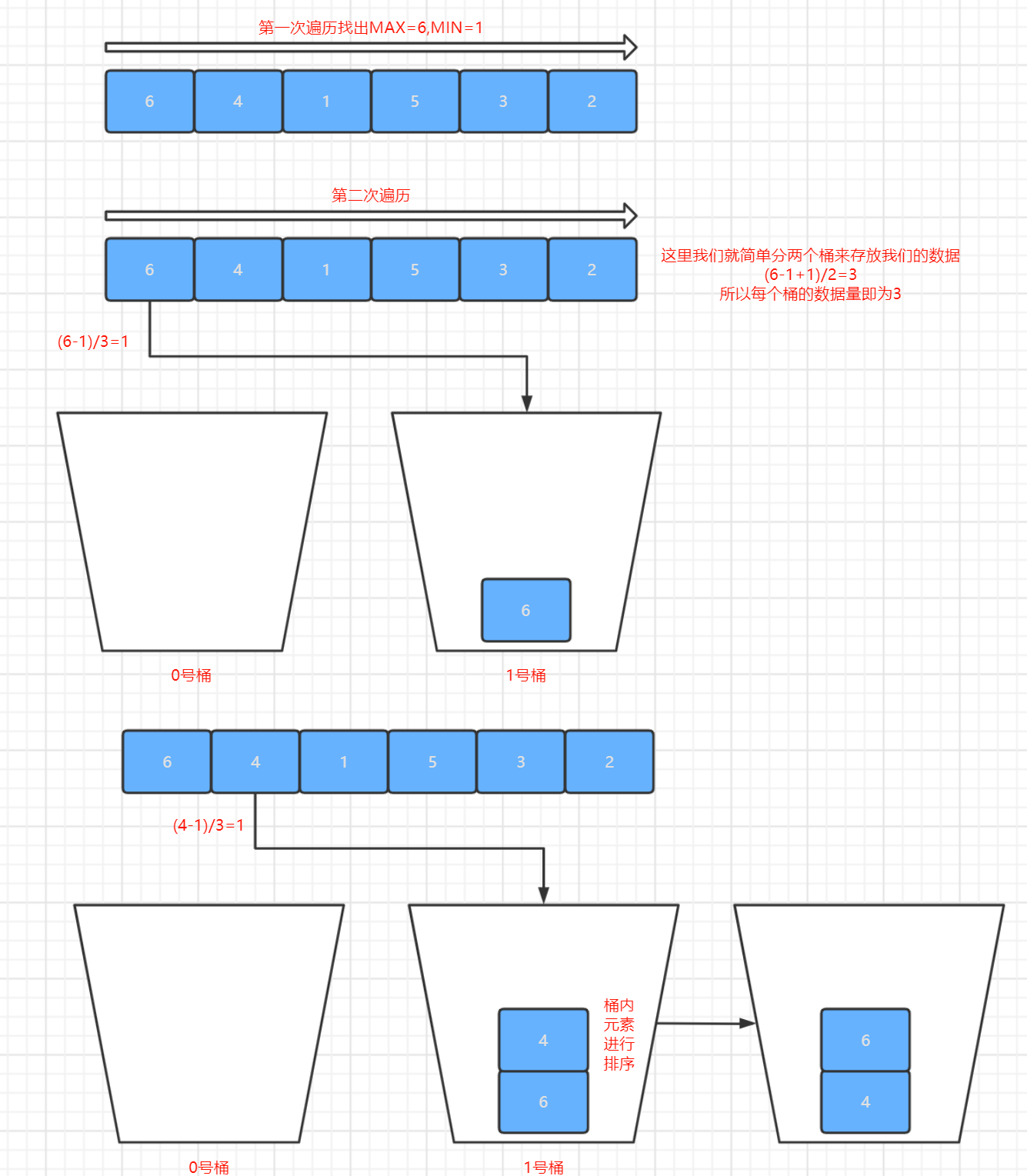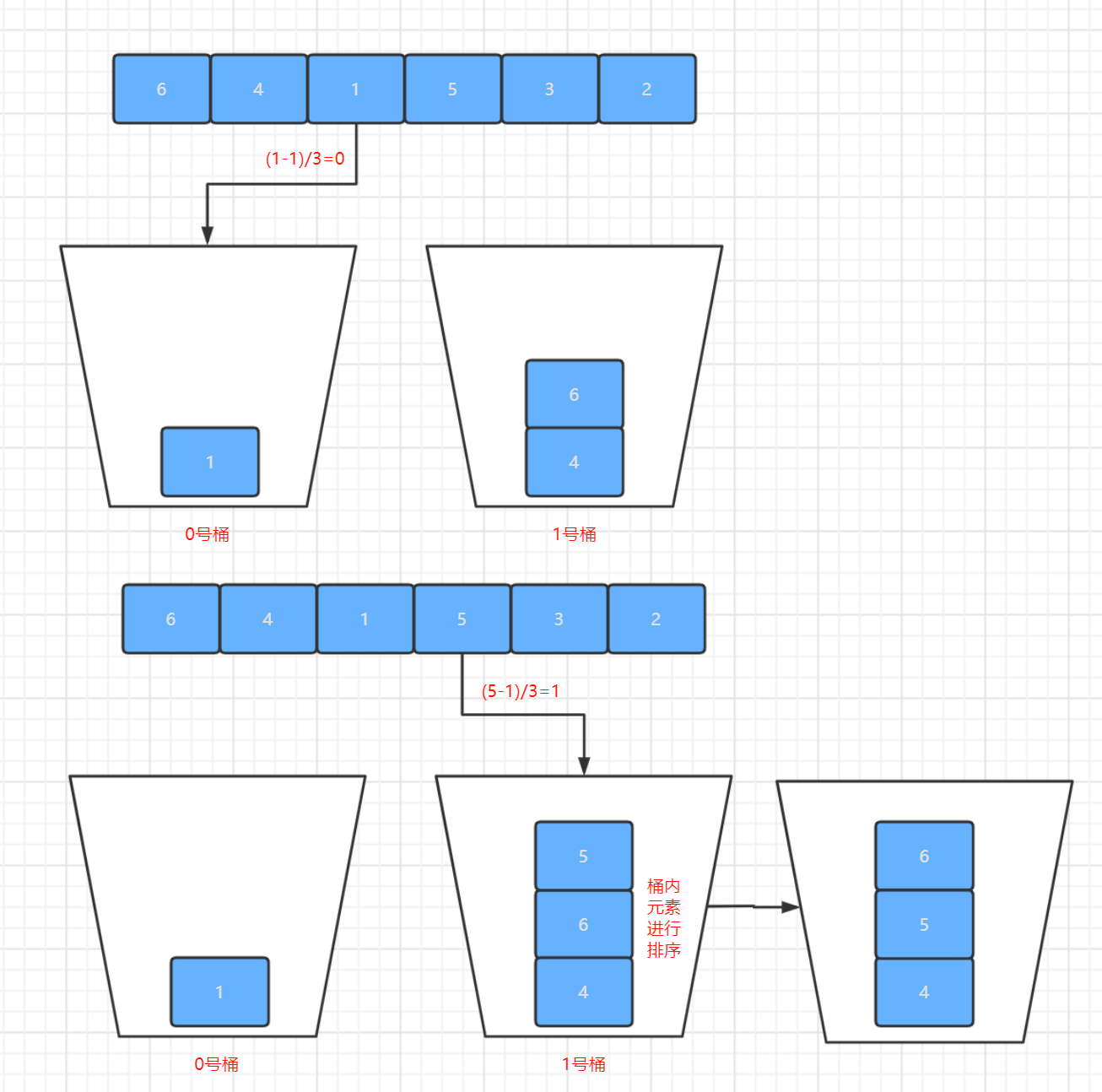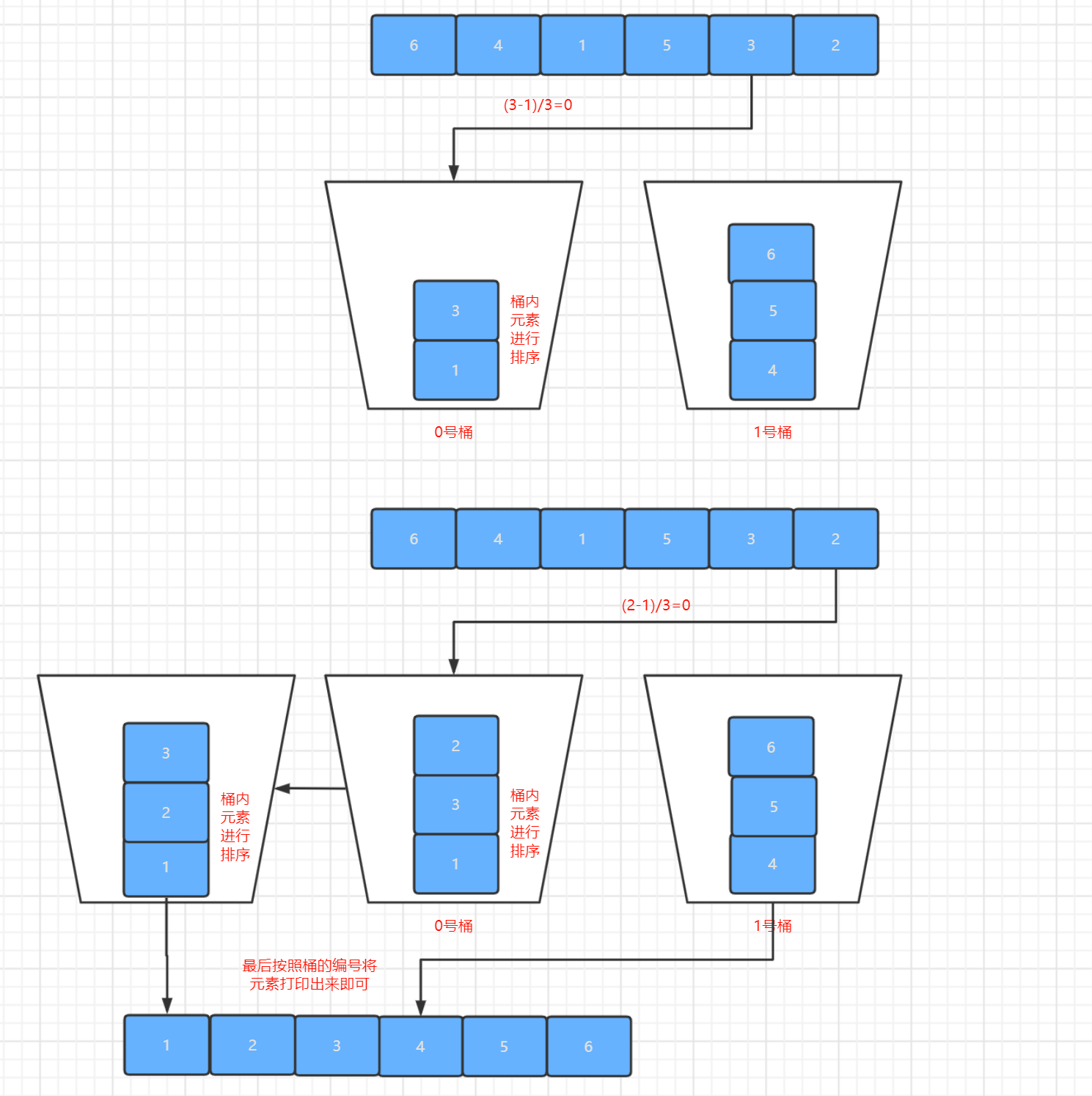• 桶排序是稳定的,原因和上面计数排序的理由是一样的.
• 桶排序也是一个通过空间换取时间的算法,但是他的空间复杂度是可以控制的.就像我们上面说的主要就是控制桶的数量.如果桶的数量设置的合理,既能降低时间复杂度,也能降低空间复杂度.//在链表中添加元素的同时需要进行元素的排序
public static void sort(ArrayList<Integer>list,int i) {
if(list==null)
//这里采用的排序方式为插入排序
else {
int flag=list.size()-1;
while(flag>=0&&list.get(flag)>i) {
if(flag+1>=list.size())
else
list.set(flag+1, list.get(flag));
flag--;
}
if(flag != (list.size()-1))
//注意这里是flag+1,自己可以尝试将这里换成flag看看,会出现数组越界的情况
list.set(flag+1, i);
else
}
}
public static void Bucketsort(int []num,int sum) {
//遍历得到数组中的最大值与最小值
int min=Integer.MAX_VALUE;
int max=Integer.MIN_VALUE;
for(int i=0;i<num.length;i++) {
min = min <= num[i] ? min: num[i];
max = max >= num[i] ? max: num[i];
}
//求出每个桶的长度,这里必须使用Double
double size=(double)(max-min+1)/sum;
ArrayList<Integer>list[]=new ArrayList[sum];
for(int i=0;i<sum;i++) {
list[i]=new ArrayList<Integer>();
}
//将每个元素放入对应的桶之中同时进行桶内元素的排序
for(int i=0;i<num.length;i++) {
System.out.println("元素:"+String.format("%-2s", num[i])+", 被分配到"+(int)Math.floor((num[i]-min)/size)+"号桶");
sort(list[(int)Math.floor((num[i]-min)/size)], num[i]);
}
System.out.println();
for(int i=0;i<sum;i++) {
System.out.println(String.format("%-1s", i)+"号桶内排序:"+list[i]);
}
System.out.println();
//顺序遍历各个桶,得出我们 已经排序号的序列
for(int i=0;i<list.length;i++) {
if(list[i]!=null){
for(int j=0;j<list[i].size();j++) {
System.out.print(list[i].get(j)+" ");
}
}
}
System.out.println();
System.out.println();
}
public static void main(String[] args) {

int []num ={7,4,9,3,2,1,8,6,5,10};
long startTime=System.currentTimeMillis();
//这里桶的数量可以你自己定义,这里我就定义成了3
Bucketsort(num, 3);
long endTime=System.currentTimeMillis();
System.out.println("程序运行时间： "+(endTime-startTime)+"ms");
}• 时间复杂度

桶排序的时间复杂度和上面的计数排序是一样的,同样也是线性级别的,但是也是增加了桶的时间,所以也是O(n+k)

• 空间复杂度

上面我们已经说过了,桶排序本身也是一个通过空间来换取时间的算法,所以很明显他的空间复杂度就会很高.并且这个空间复杂度主要就取决于桶的数量以及桶的范围,所以假设有k个桶的话,那么空间复杂度就为O(n+k)

4-基数排序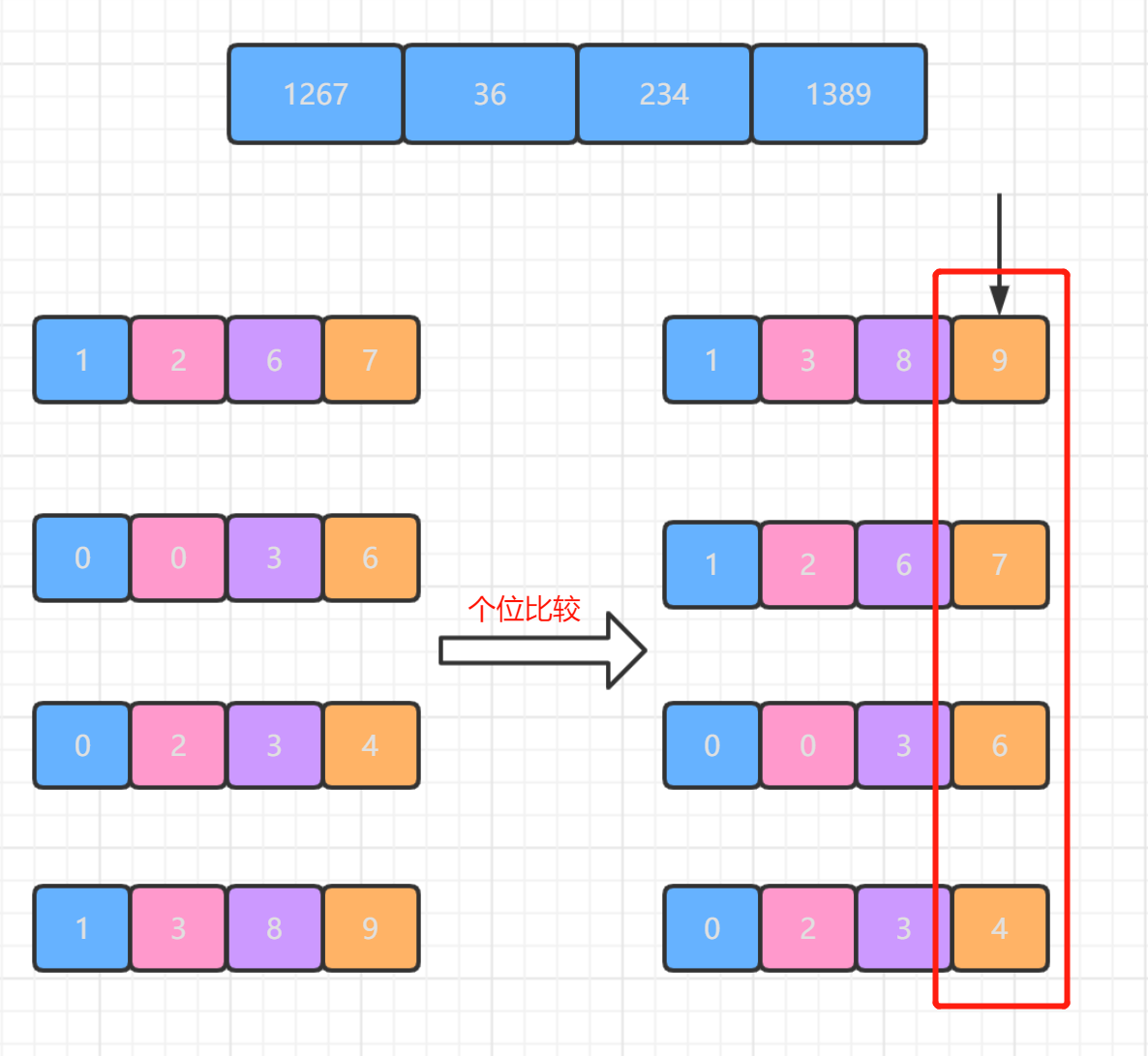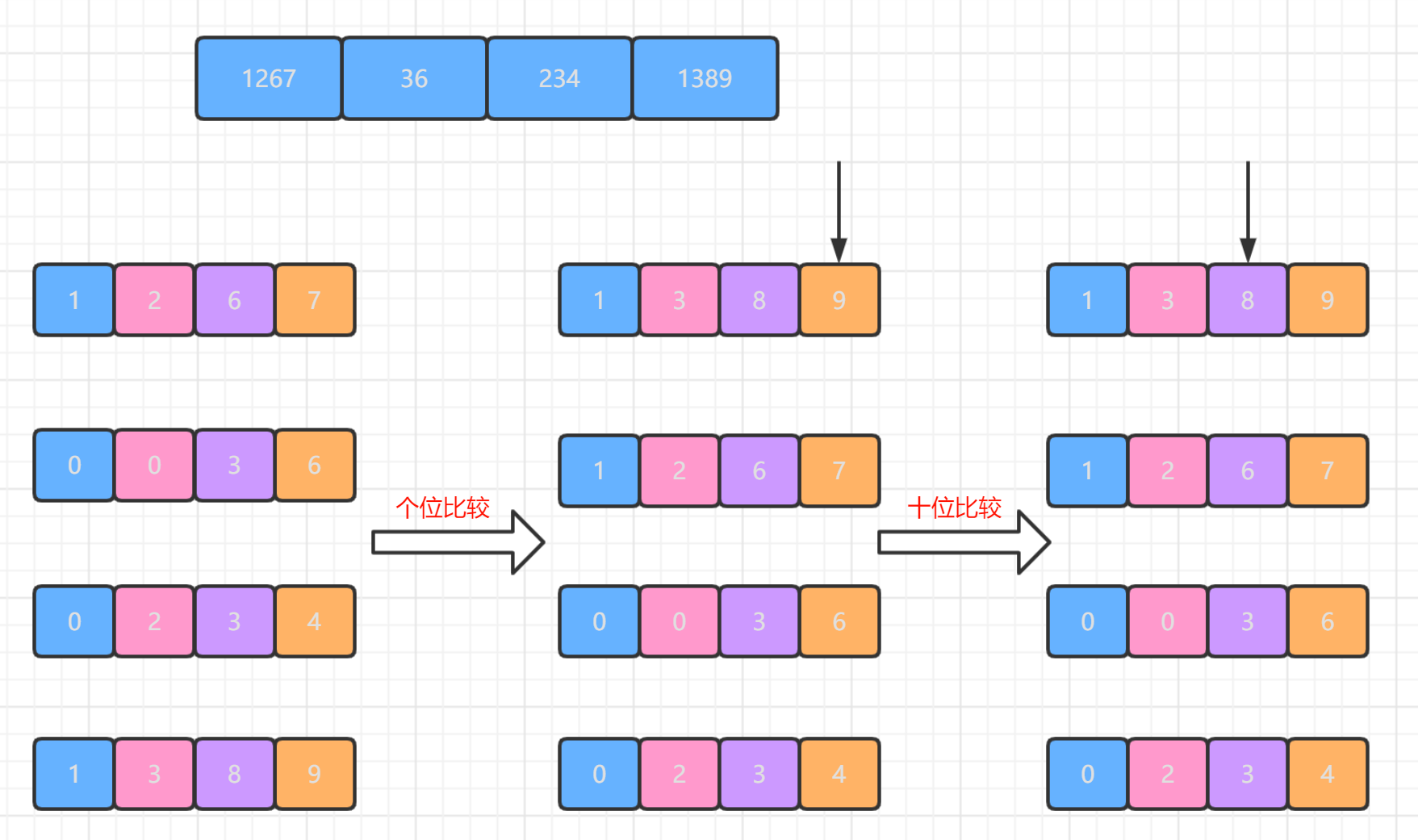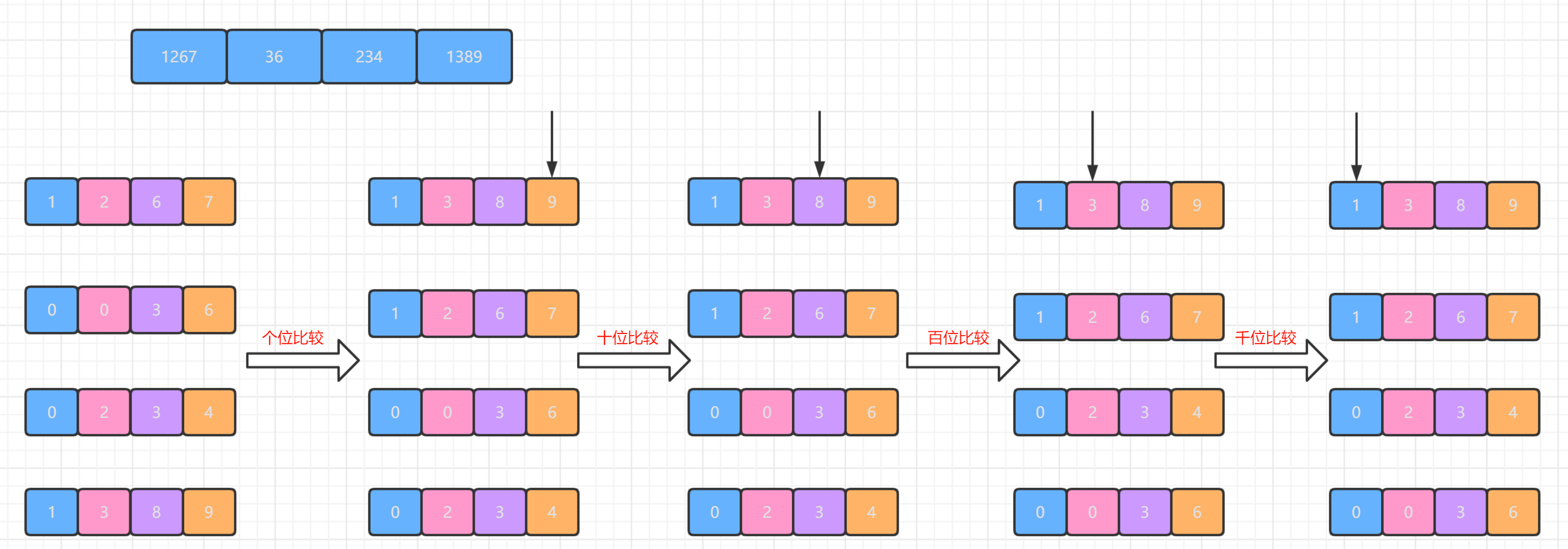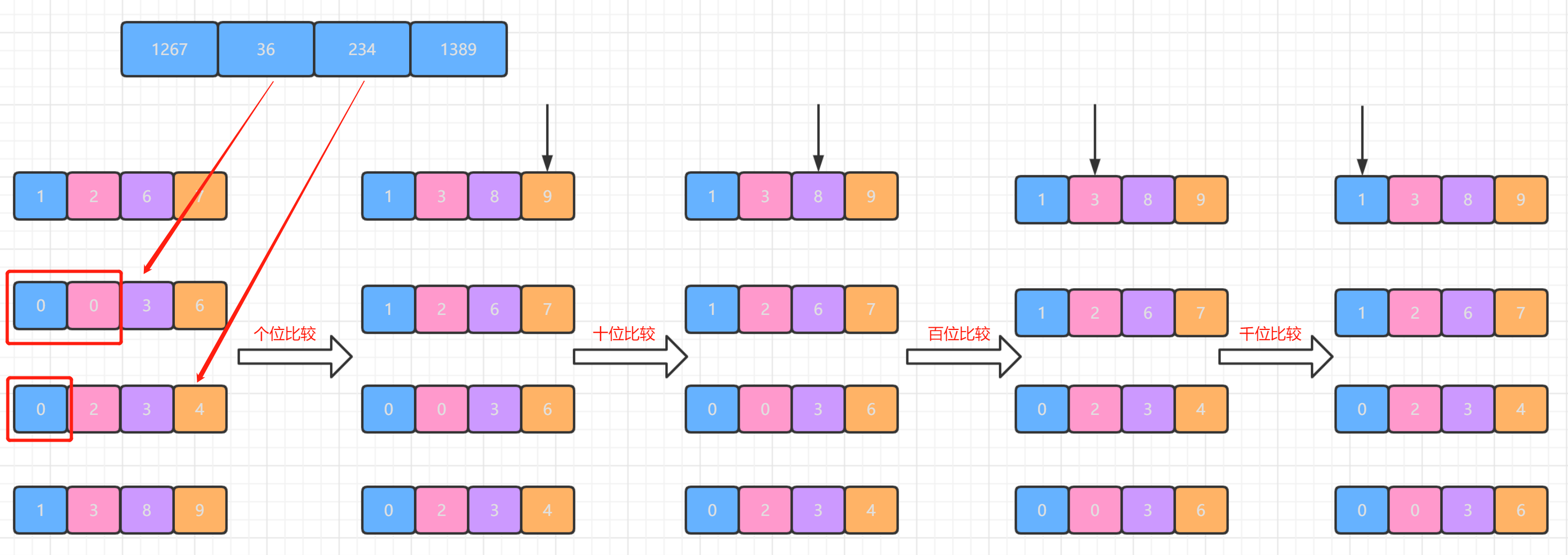• 从高位到低位进行比较

329839657839
457720457329
657657355657
839457839457
436436436436
720329720355
355355329720
• 从低位到高位进行比较

329720720329
457355329355
657436436436
839457839457
436657355657
720329451720
355839657839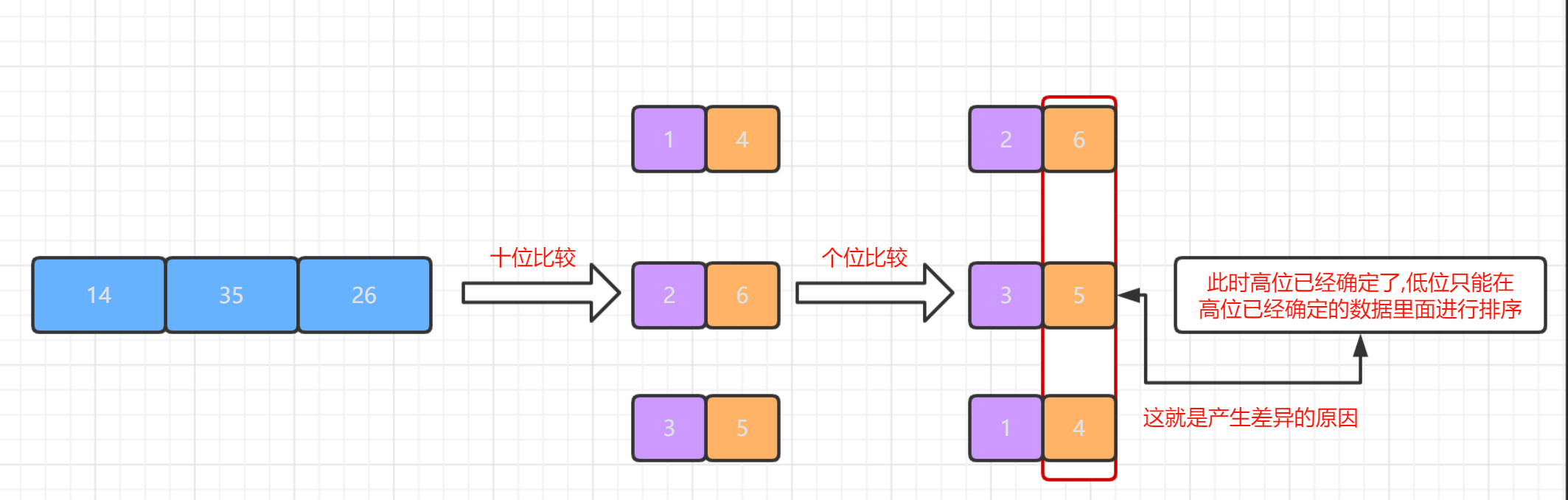• 1.我们第一次遍历序列.找出序列中的最大值MAX,找到MAX之后我们可以确定我们需要比较多少数位了.

• 2.这时候我们就需要按照元素在该位数对应的数字将元素存入到相应的容器之中.如下图所示: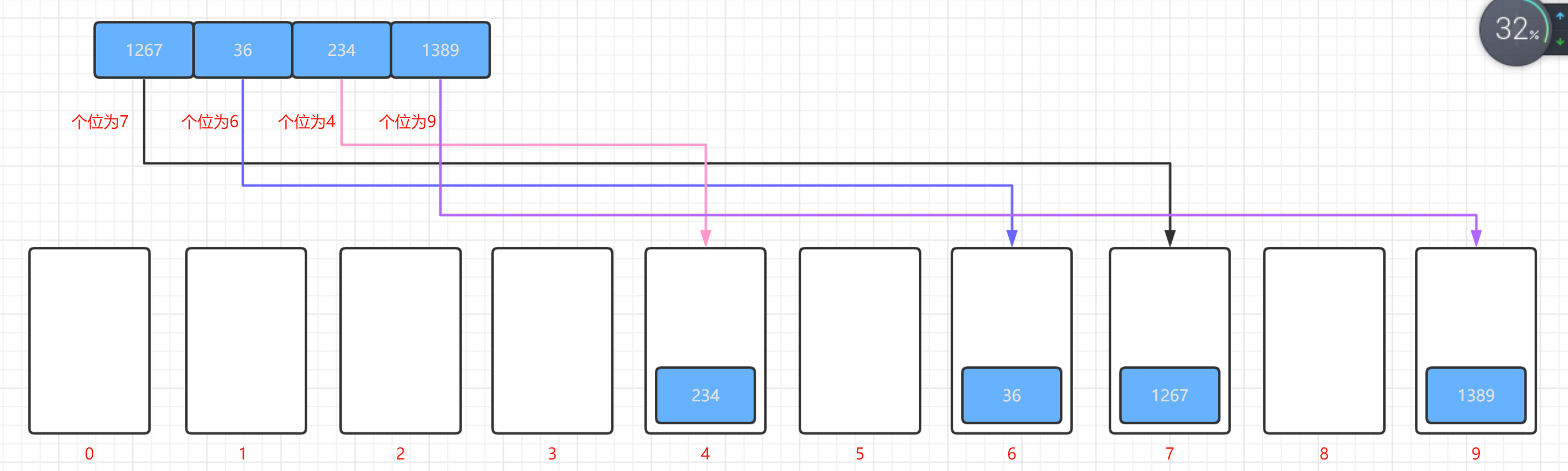• 3.之后我们再按照容器的顺序将元素重新弹出构成我们接下来需要排序的序列,如下图所示: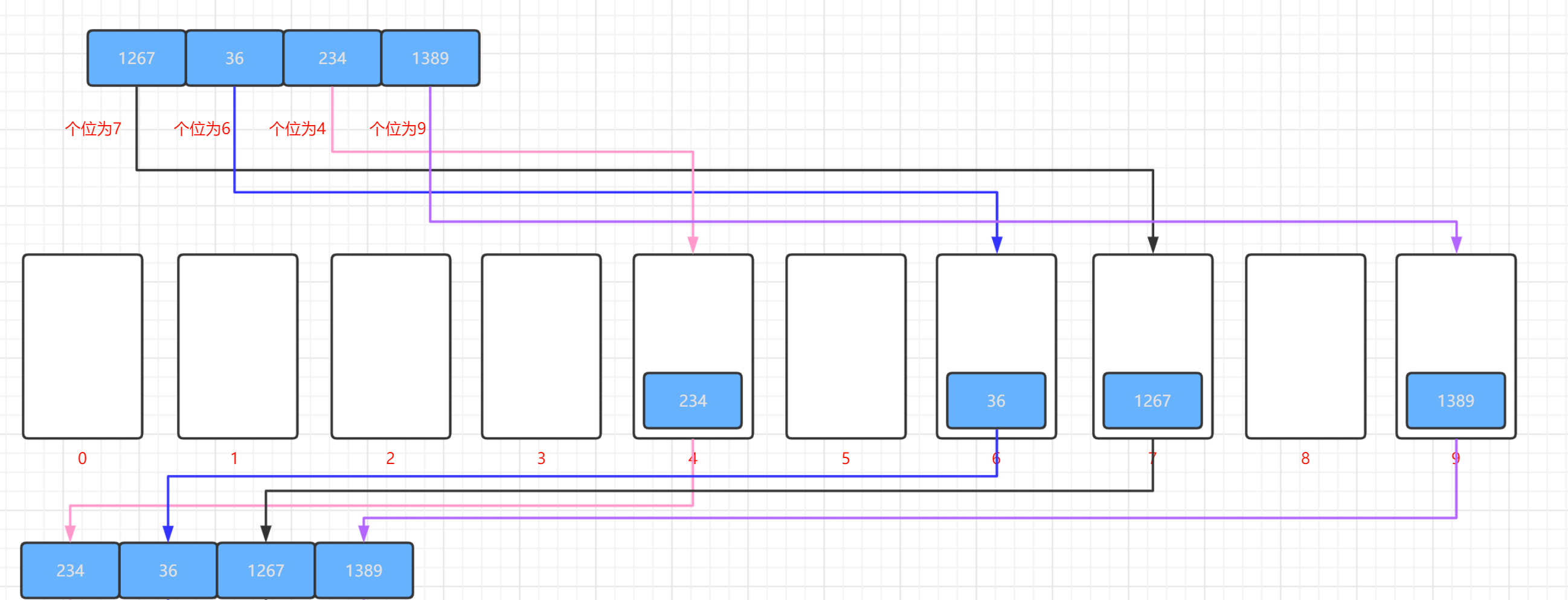这个从容器弹出的过程需要注意一点,那就是遵循先进先出的原则,所以这个容器选择队列或者是链表比较合适,不能选择栈,因为栈是先进后出,拿取元素的时候回非常麻烦.

• 4.最后只需要重复2,3步骤,直到最高位也比较完毕,那么整个基数排序就已经完成了.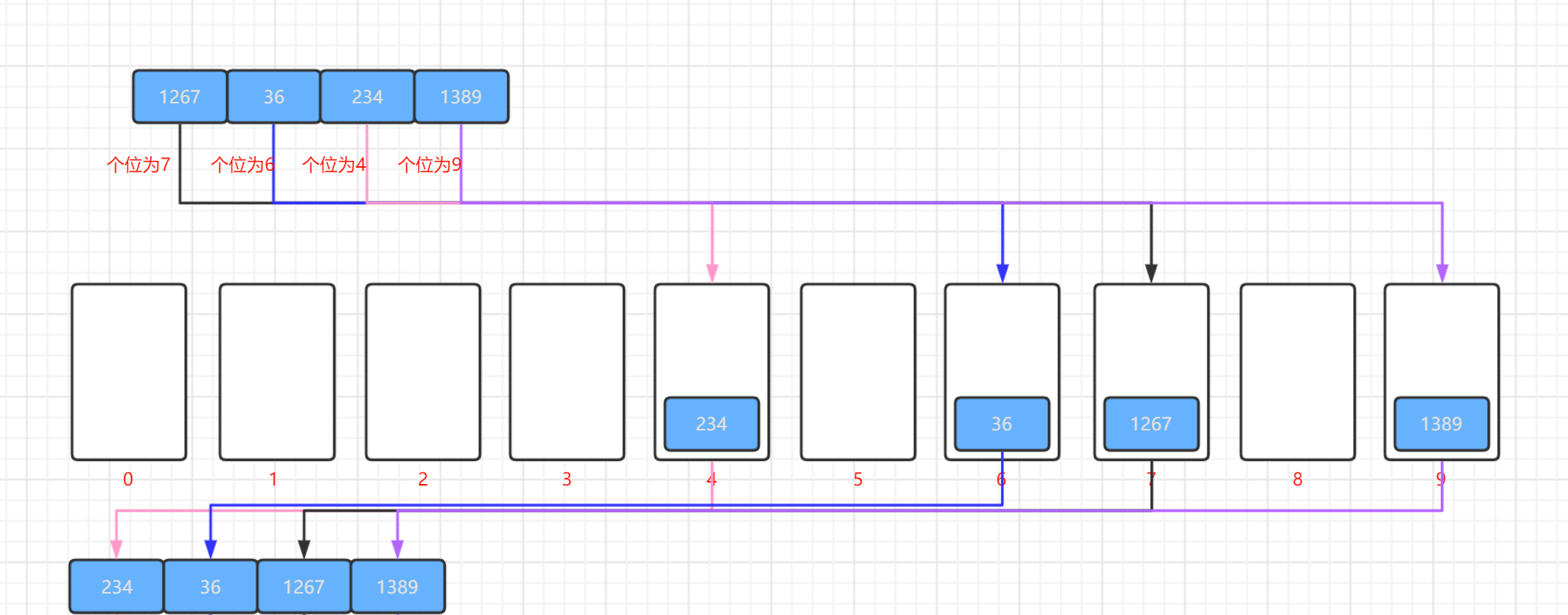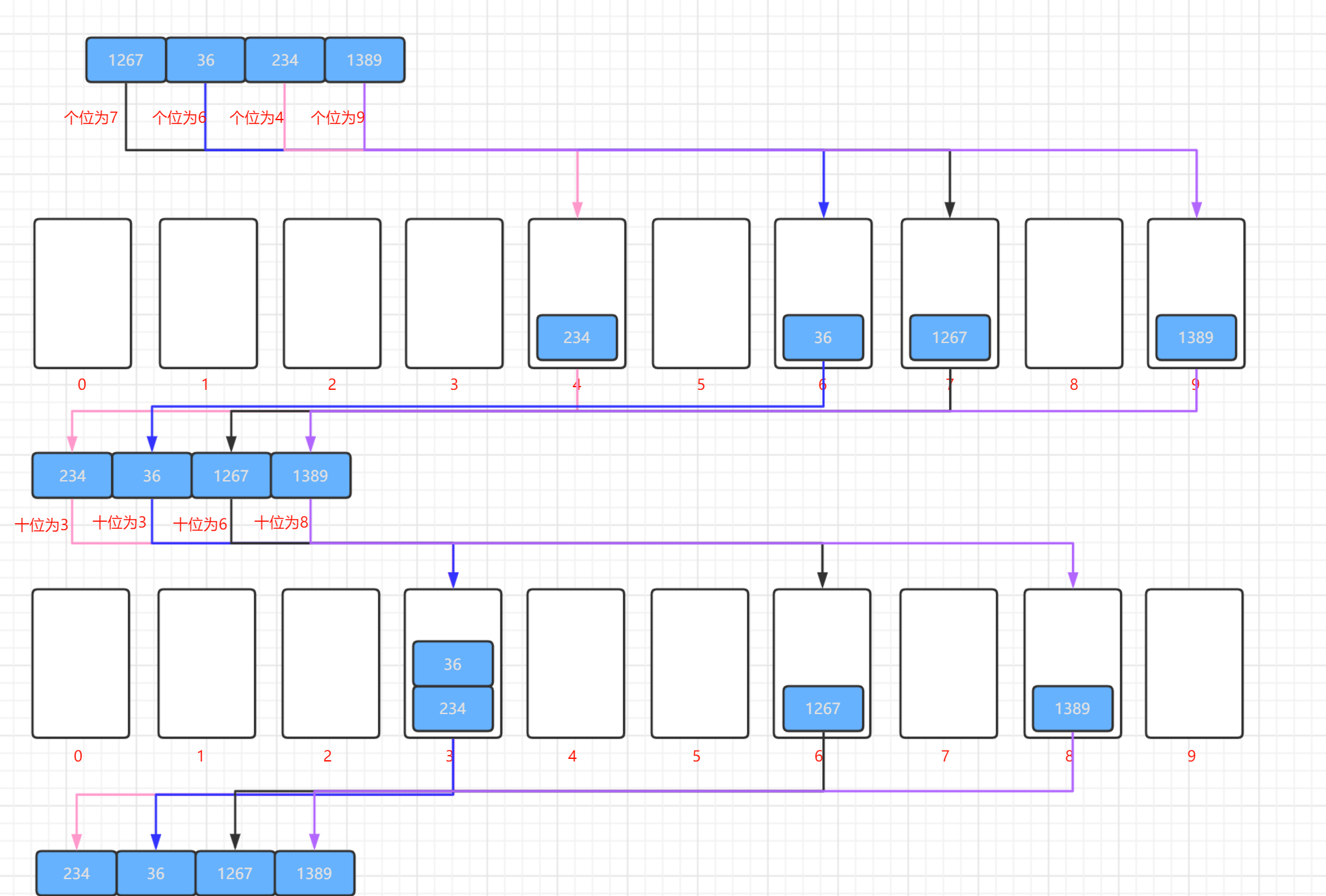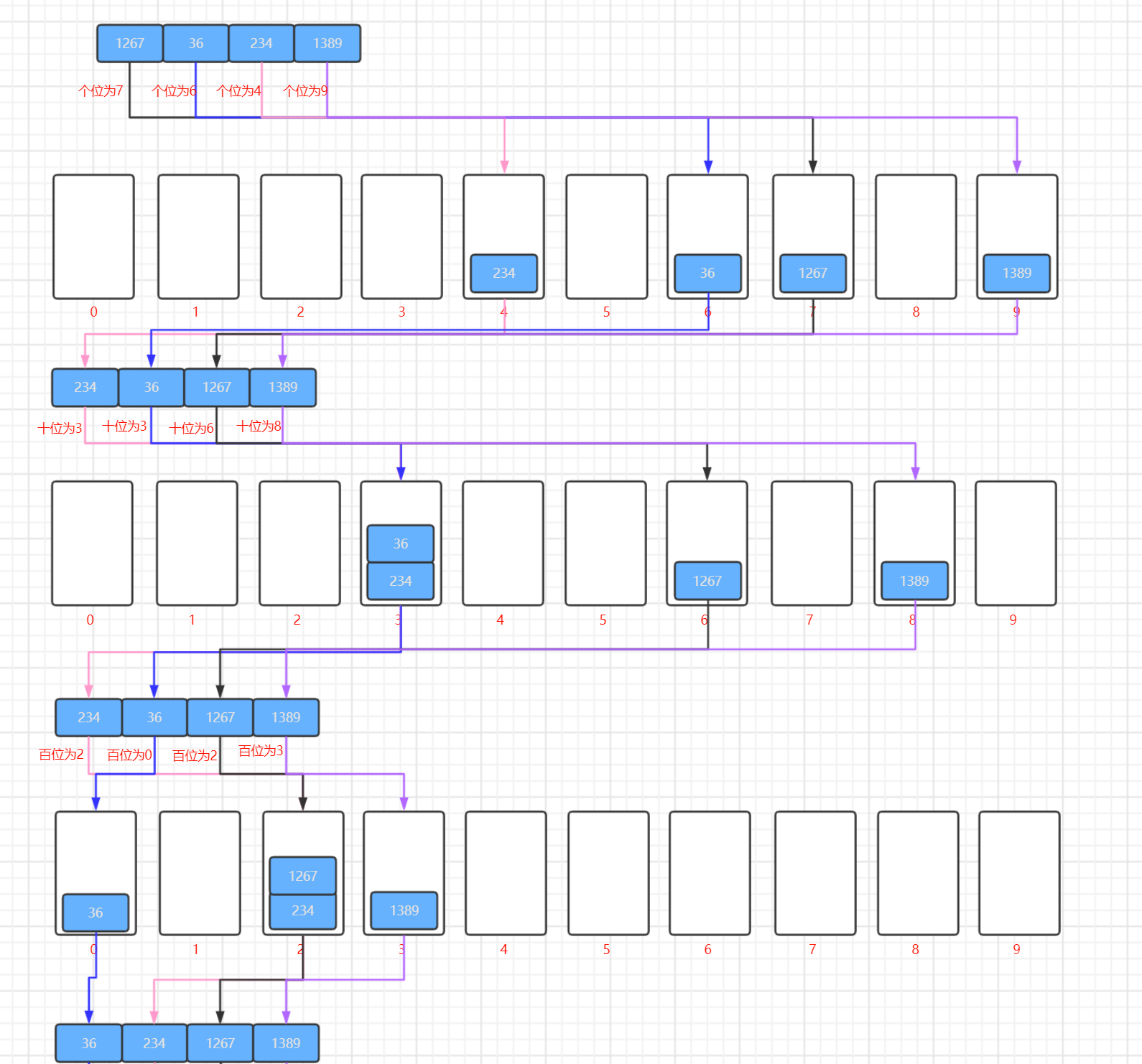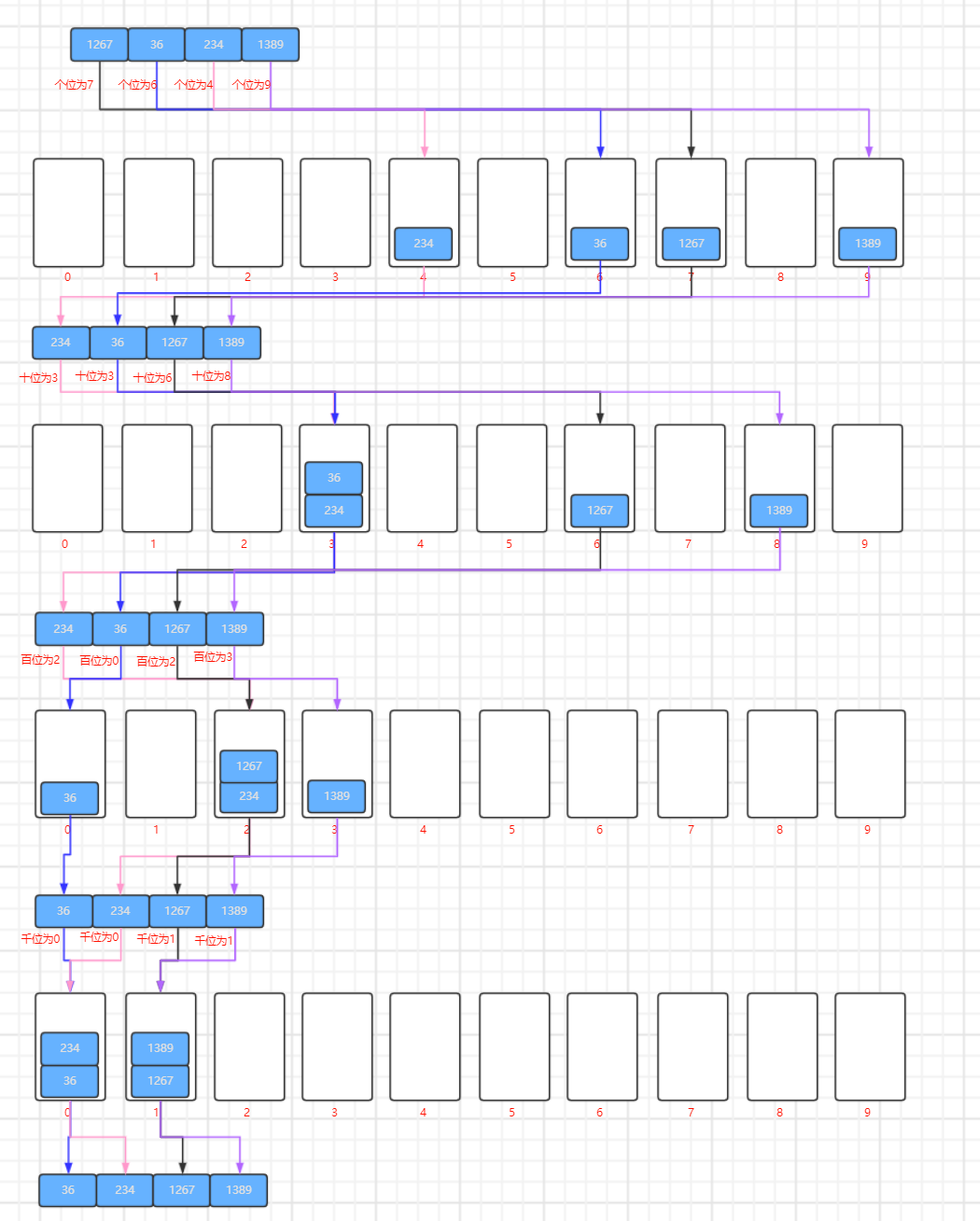• 基数排序是稳定的,原因和桶排序以及计数排序的原因一样.//将所有的数组合并成原来的数组
public static void merge(ArrayList<Integer> list[],int num[]) {
int k=0;
for(int i=0;i<list.length;i++) {
if(list[i]!=null) {
for(int j=0;j<list[i].size();j++) {
num[k++]=list[i].get(j);
System.out.print(num[k-1]+" ");
}
}
//合并完成之后需要将链表清空,否则元素会越来越多
list[i].clear();
}
System.out.println();
}
//将所有的元素分散到各个链表之中
public static void split(ArrayList<Integer> list[],int num[],int k) {
for(int j=0;j<num.length;j++) {
}
System.out.println("-----------------------------------------------------------------------");
System.out.println("个位开始数,第"+(String.valueOf(k).length())+"位排序结果:");
for(int j=0;j<10;j++) {
System.out.println((String.valueOf(k).length())+"号位,数值为"+j+"的链表结果:"+list[j]);
}
}
public static void main(String[] args) {
ArrayList<Integer>list[]=new ArrayList ;
for(int i=0;i<10;i++) {
list[i]=new ArrayList<Integer>();
}
int []num ={7,14,9,333,201,1,88,6,57,10,56,74,36,234,456};
long startTime=System.currentTimeMillis();
int max=Integer.MIN_VALUE;
//第一次遍历获得序列中的最大值
for(int i=0;i<num.length;i++) {
if(num[i]>max)
max=num[i];
}
int k=1;
for(int i=0;i<String.valueOf(max).length();i++) {
split(list, num, k);
System.out.println("第"+(i+1)+"次排序");
merge(list, num);
k=k*10;
}
long endTime=System.currentTimeMillis();
System.out.println("程序运行时间： "+(endTime-startTime)+"ms");
}• 时间复杂度

看完我们上面的动图演示之后我们可以看到基数排序只需要根据最大元素的位数,遍历相应次数的序列即可,所以我们可以确定基数排序的时间复杂度一定是线性级别的,但是虽然是线性级别的,但是有一个系数的,这个系数就是最大元素的位数K,所以时间复杂度应该是O(n*k)

• 空间复杂度

空间复杂度我们也可以看出来,主要就是取决于链表的数量以及序列元素的数量,所以空间复杂度为O(n+k)01-265852
05-181万+

04-016万+
03-18346
08-25344
05-221066
08-04451
05-07162
08-09399
03-163758
07-18276
11-041311
05-0863
03-051783
10-11526
05-02584
05-22188
08-081万+
06-1471¥2 ¥4 ¥6 ¥10 ¥20余额支付 (余额：-- )扫码支付获取中扫码支付点击重新获取扫码支付1.余额是钱包充值的虚拟货币，按照1:1的比例进行支付金额的抵扣。
2.余额无法直接购买下载，可以购买VIP、C币套餐、付费专栏及课程。余额充值